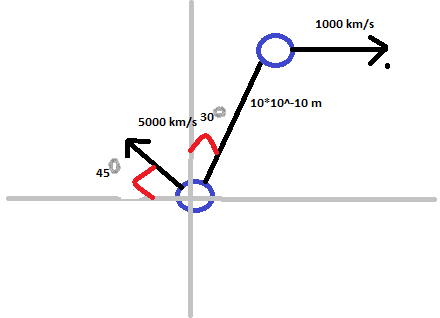Two Point Charges and Magnetic Field

Homework Statement

Find the magnetic forces acting on each electron in the attached imageHomework Equations

B = $\frac{μ0}{4pi}$*q*$\frac{v x \hat{r}}{r^{2}}$
F$_{m}$ = qv x B

The Attempt at a Solution

B$_{1}$ = 10$^{-7}$ * 1.6*10$^{-19}$ * $\frac{5000*10^{3}*sin(45)}{(10*10^{-10})(10*10^{-10})}$
B$_{1}$ = .057

Fm$_{2}$ = (1.6*10$^{-19}$)*1000*10^3*.057
Fm$_{2}$ = 9.12*10^{-15}

Now at this point I know something is wrong, but I'm not sure what. I thought the force on the 2nd electron would be the force created by the 1st electron, but that number is definitely screaming incorrect to me. I figure it I understand how to figure out the force on 1 I can figure out the force on the other. (Electron 1 is at the bottom, electron 2 at the top). Where is my mistake?

Attachments

• New Bitmap Image.bmp
410 KB · Views: 407
Last edited:

Woops, don't try and do physics half asleep!Those first two errors aside, I think I see what angle I am supposed to use. The velocity of B$_{1}$ is 45° from the x axis, but 75° from $\hat{r}$, since $\hat{r}$ is along r, correct?